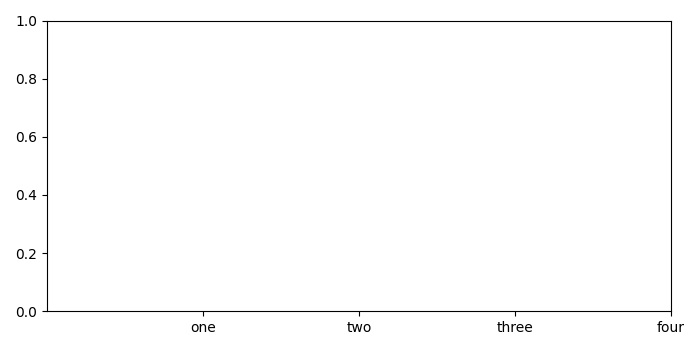# Getting empty tick labels before showing a plot in Matplotlib

To get empty tick labels before showing a plot in matplotlib, we can take the following Steps −

• Create a list of data points.

• Add a subplot to the current figure using subplot() method.

• Set ticks and ticklabels using set_xticks() method and set_xticklabels() method.

• To get the empty tick labels, use get_xticklabels(which='minor').

• To display the method, use show() method.

## Example

from matplotlib import pyplot as plt
plt.rcParams["figure.figsize"] = [7.00, 3.50]
plt.rcParams["figure.autolayout"] = True
x = [1, 2, 3, 4]
ax1 = plt.subplot()
ax1.set_xticks(x)
ax1.set_xticklabels(["one", "two", "three", "four"])
print("Empty tick labels: ", ax1.get_xticklabels(which='minor'))
plt.show()

## OutputUpdated on: 15-May-2021

500 Views